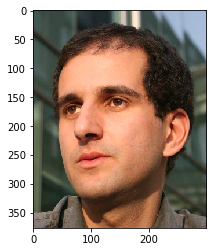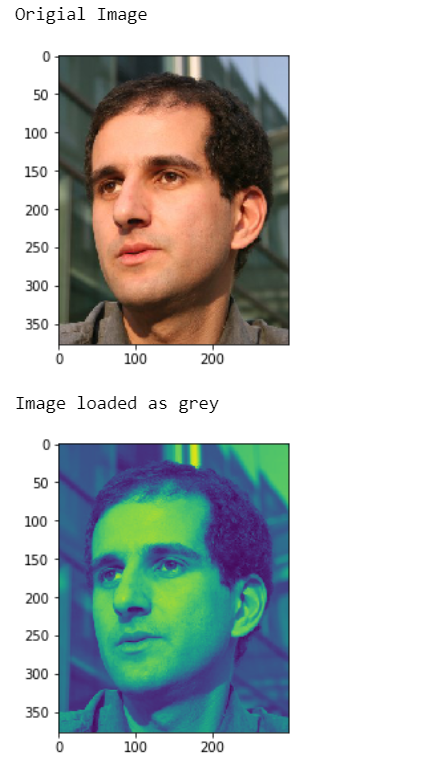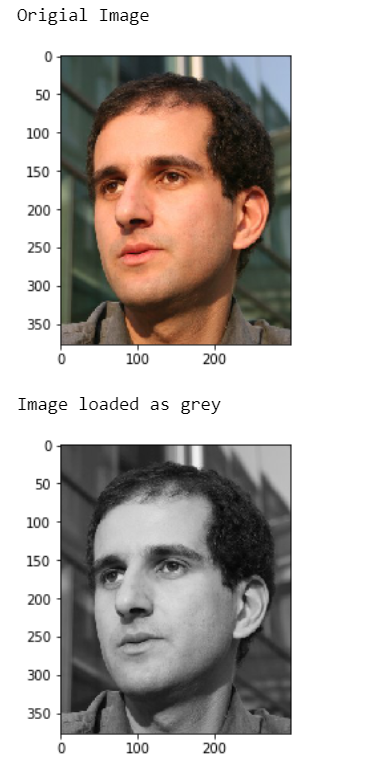In this article we will see how we can load the image as grey in mahotas, there are lots of images available in mahotas we use mahotas.demos.load method to load them, in this tutorial we will use “luispedro” image, below is the command to load it.

`mahotas.demos.load('luispedro')`

Below is the luispedro imageIn order to do this we will use mahotas.demos.load method

Argument : It takes image name as argument
Return : It returns numpy.ndarray i.e image object

Example 1:

## Python3

 `# importing required libraries` `import` `mahotas` `import` `mahotas.demos` `import` `numpy as np` `from` `pylab ``import` `imshow, gray, show` `from` `os ``import` `path`   `# loading the image` `photo ``=` `mahotas.demos.load(``'luispedro'``)`   `# showing original image` `print``(``"Original Image"``)` `imshow(photo)` `show()`   `# loading image as grey` `photo ``=` `mahotas.demos.load(``'luispedro'``, as_grey ``=` `True``)`   `# showing image` `print``(``"Image loaded as grey"``)` `imshow(photo)` `show()`

Output :Example 2:

## Python3

 `# importing required libraries` `import` `mahotas` `import` `mahotas.demos` `import` `numpy as np` `from` `pylab ``import` `imshow, gray, show` `from` `os ``import` `path`   `# loading the image` `photo ``=` `mahotas.demos.load(``'luispedro'``)`   `# showing original image` `print``(``"Original Image"``)` `imshow(photo)` `show()`   `# loading image as grey` `photo ``=` `mahotas.demos.load(``'luispedro'``, as_grey ``=` `True``)`   `# converting image type to unit8` `# because as_grey returns floating values` `photo ``=` `photo.astype(np.uint8)`   `# calling gray method` `gray()`   `# showing image` `print``(``"Image loaded as grey"``)` `imshow(photo)` `show()`

Output :Whether you're preparing for your first job interview or aiming to upskill in this ever-evolving tech landscape, GeeksforGeeks Courses are your key to success. We provide top-quality content at affordable prices, all geared towards accelerating your growth in a time-bound manner. Join the millions we've already empowered, and we're here to do the same for you. Don't miss out - check it out now!